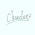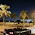## Wednesday, 20 June 2012

### Remainder Theorem

1. According to Remainder Theorem: Let p(x) be any polynomial and a be any real number. If p(x) is divided by the linear polynomial x - a, then the remainder is p(a).

2. If p(x) is divided by  (x + a), then remainder is p(-a).

3. If p(x) is divided by (ax -b) then remainder is p(b/a).

4. If p(x) is divided by (ax + b), then remainder is p(-b/a).

5. In above cases, -a, b/a and -b/a are the zeros of the divisors x + a, ax-b and ax+b respectively.

Exercise 2.3

Q1: Find the remainder when x3 + 3x2 + 3x + 1 is divided by
(i)   x + 1
(ii)  x - 1/2
(iii) x
(iv) x + π
(v) 5 + 2x

Answer:  The remainder of the polynomial can be found by following methods:
• long division method
• applying remainder theorem.
• synthetic division (not covered here)
(i) x + 1
By long division method,
```        x2 + 2x + 1
____________________________
x+1) x3 + 3x2 + 3x + 1
x3 + x2
-   -
――――――――――
2x2 + 3x
2x2 + 2x
-    -
――――――――――
x + 1
x + 1
-  -
――――――――
0 ```

Therefore remainder is 0.
IInd Method:  q(x) = x3 + 3x2 + 3x + 1
If t(x) = x+1 is the divisor of the polynomial, by remainder theorem,   remainder is q(-1)
q(-1) = (-1)3 + 3(-1)2 + 3(-1) + 1 = -1 + 1 -1 +1 = 0.
∴ remainder is 0.

(ii) x - 1/2
p(x) =  x3 + 3x2 + 3x + 1
Zero of x-1/2 = 1/2
By remainder theorem, when p(x) is divided by x-1/2, the remainder is p(1/2).
∴  p(1/2) = (1/2)3 + 3(1/2)2 + 3(1/2) + 1
= 1/8 + 3/4 + 3/2 + 1 = 27/8

(iii) x
Zero of x is 0
∴ p(0) = (0)3 + 3(0)2 + 3(0) + 1 = 1

So remainder is 1.

(iv) x + π
Root of   x + π is  (x + π = 0 ⇒ x = -π)  = -π
By remainder theorem, if -π divides p(x)then remainder is p(-π) i.e.
p(-π) = (-π)3 + 3(-π)2 + 3(-π) + 1 = -π3 + 3π2 - 3π + 1  ... (answer)

(v)  5 + 2x
Root of 5 + 2x is -5/2
If -5/2 divides p(x) =  x3 + 3x2 + 3x + 1, by remainder theorem the remainder is p(-5/2)
∴ p(-5/2) = (-5/2)3 + 3(-5/2)2 + 3(-5/2 + 1
= -125/8  + 3(25/4) -15/2 + 1
= -125/8 + 75/4 - 15/2 + 1
= -27/8

Q2: Find the remainder when x3 – ax2  + 6x – a is divided by x – a.

Answer: By remainder theorem, if (x = a) divides  p(x) = x3 – ax2  + 6x – a then remainder of polynomial is p(a).
∴p(a) = a3 – a(a)2  + 6a – a  = a3 – a3  + 6a – a  = 5a  ...(answer)

As an alternate method you may find remainder by long division method.

Q3:  Check whether 7 + 3x is a factor of 3x3 + 7x.

Answer: Zero of 7 + 3x is:
7+ 3x = 0  ⇒ 3x = -7   ⇒ x = -7/3
If  p(x) = 3x3 + 7x. is divisible by (7 + 3x) then its remainder is zero i.e. ⇔ p(-7/3) = 0
p(-7/3) = 3(-7/3)3 + 7(-7/3) = 3(-343/27) - 49/3
= -343/9 -49/3 = -490/9 ≠ 0
⇒ 7 + 3x is not a factor of 3x3 + 7x

Q4:  Prove Remainder theorem.

AnswerRemainder theorem: Let p(x) be any polynomial of degree ≥ 1, and 'a' is any real number. If p(x) is divided by (x-a) then remainder is p(a).

Proof: Suppose q(x) is the quotient and r(x) is the remainder, when (x-a) divides p(x).
Since  Dividend = Divisor ✕ Quotient + Remainder.

Similarly we can have,
p(x) = (x-a) ✕ q(x) + r(x)                    ...(I)

Where r = 0 or degree of r(x) < degree of (x -a).
Since (x-a) is a linear equation. The degree of (x-a) is 1  and degree of r(x) is less than the degree of x-a, ⇒ the degree of r(x) = 0.

It means r(x) is a constant, say r. ∴ Eqn. I will become:
p(x) = (x-a) ✕ q(x) + c

Replacing x by a i.e. x =a , we have

p(a) = (a-a) ✕ q(x) + c = 0 + c = c
⇒p(a) = c  which proves the remainder theorem.

Q5: 6x2 + ax + 7 when divided by x-2 gives remainder 13. Find the value of a.

Answer: Let p(x) = 6x2 + ax + 7.
According to remainder theorem, if (x-2) divides p(x) and remainder is 13 then, p(2) =  13
p(2) = 6(2)2 + a(2) + 7 = 13
⇒   24 + 2a + 7 = 13
⇒  2a = -24-7+13 = -18
⇒ a = -9

Q6: The polynomial x4 - 2x3 + 3x2 - ax + b when divided by (x+1) and (x-1) gives remainders 19 and 5 respectively. Find the remainder when the polynomial is divided by (x-3).

Answer: Let p(x) =  x4 - 2x3 + 3x2 - ax + b
Writing the divisors in (x -a) form i.e. (x-(-1)) and (x-1).

According to  remainder theorem, if (x-a) divides the polynomial p(x) and c is the remainder, then p(a) =c.

∴  p(-1) = 19   and p(1) = 5
⇒  p(-1) = (-1)4 - 2(-1)3 + 3(-1)2 - a(-1) + b = 19
⇒  1 - 2(-1) + 3 + a + b = 19
⇒  1 + 2 + 3 + a + b = 19
⇒  a + b = 13                                                 ...(I)

and p(1) = (1)4 - 2(1)3 + 3(1)2 - a(1) + b =5
⇒  1 - 2 + 3 -a + b = 5
⇒   -a + b = 3                                                ...(II)

Adding equation I and II, gives
2b = 16   ⇒   b = 8
and  a = 5

∴   p(x) = x4 - 2x3 + 3x2 - 5x + 8

The divisor is (x-3). Applying remainder theorem, remainder = p(3).
i.e. p(3) =  (3)4 - 2(3)3 + 3(3)2 - 5(3) + 8 = 81 - 54 + 27 - 15 + 8 = 47     ...(answer)

Watch KhanAcademy's (Wonderful Elearning site) Video on Long Division:1.Class IX Maths chapter 3,9,10,11,12 are missing please add them as fast as u can

2.thx a lot to the site..love gyz

3.thx a lot to the site..love gyz

4.Great site can you simplify these a bit
Call vivek sir for further clarification 9995693694